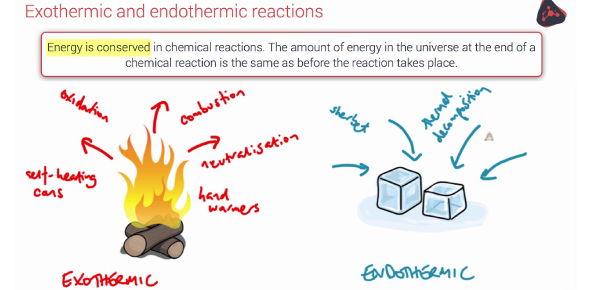# Endothermic And Exothermic Chemical Reactions! Trivia Quiz

10 Questions | Total Attempts: 3621SettingsEndothermic and Exothermic are chemical reactions that absorb and release heat. An example of an endothermic reaction is photosynthesis. An exemplar of an exothermic reaction is combustion. Specifically, exothermic reactions are those in which heat is given out, while with endothermic reactions, heat is taken in. For this quiz, you will be provided with chemical formulas, and it is up to you to determine its type of reaction. Stay cool and don’t overheat; you will ACE this quiz.

• 1.
C6H12O6    +  6O2   à  6CO2   +    6H2O    ∆H  =  -2803 kJ
• A.

Exothermic

• B.

Endothermic

• 2.
6CO2   +    6H2O    à  C6H12O6    +  6O2    ∆H  =  +2803 kJ
• A.

Exothermic

• B.

Endothermic

• 3.
3NO2 +   H2O  à  2HNO3   +  NO  ∆H  =  - 138 kJ
• A.

Exothermic

• B.

Endothermic

• 4.
C  +  H2O  à  CO  + H2      ∆Hrxn = + 113 kJ
• A.

Exothermic

• B.

Endothermic

• 5.
CH4 + 2 O2 --> CO2 + 2 H20 + 213.2 KJ
• A.

Exothermic

• B.

Endothermic

• 6.
N2(g) + O2(g) + 43 kcal -----> 2 NO(g)
• A.

Exothermic

• B.

Endothermic

• 7.
• A.

Exothermic

• B.

Endothermic

• 8.
C3H8(g) + 5 O2(g) à 3 CO2(g) + 4H2O(g) + 2200 kJ
• A.

Exothermic

• B.

Endothermic

• 9.
• A.

Exothermic

• B.

Endothermic

• 10.
If a reaction finishes with more energy than it started, it must be a
• A.

Exothermic

• B.

Endothermic

Related TopicsBack to top